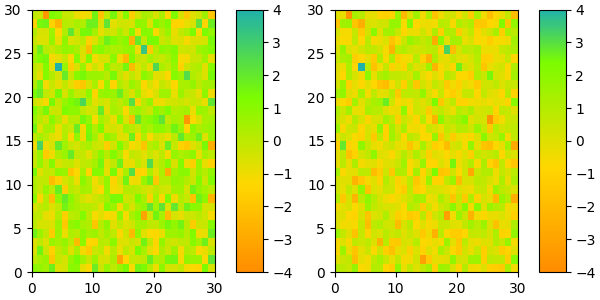# Creating Colormaps in Matplotlib¶

Matplotlib has a number of built-in colormaps accessible via matplotlib.cm.get_cmap. There are also external libraries like palettable that have many extra colormaps.

However, we often want to create or manipulate colormaps in Matplotlib. This can be done using the class ListedColormap or LinearSegmentedColormap. Seen from the outside, both colormap classes map values between 0 and 1 to a bunch of colors. There are, however, slight differences, some of which are shown in the following.

Before manually creating or manipulating colormaps, let us first see how we can obtain colormaps and their colors from existing colormap classes.

## Getting colormaps and accessing their values¶

First, getting a named colormap, most of which are listed in Choosing Colormaps in Matplotlib, may be done using matplotlib.cm.get_cmap, which returns a colormap object. The second argument gives the size of the list of colors used to define the colormap, and below we use a modest value of 8 so there are not a lot of values to look at.

import numpy as np
import matplotlib.pyplot as plt
from matplotlib import cm
from matplotlib.colors import ListedColormap, LinearSegmentedColormap

viridis = cm.get_cmap('viridis', 8)


The object viridis is a callable, that when passed a float between 0 and 1 returns an RGBA value from the colormap:

print(viridis(0.56))


Out:

(0.122312, 0.633153, 0.530398, 1.0)


### ListedColormap¶

ListedColormap s store their color values in a .colors attribute. The list of colors that comprise the colormap can be directly accessed using the colors property, or it can be accessed indirectly by calling viridis with an array of values matching the length of the colormap. Note that the returned list is in the form of an RGBA Nx4 array, where N is the length of the colormap.

print('viridis.colors', viridis.colors)
print('viridis(range(8))', viridis(range(8)))
print('viridis(np.linspace(0, 1, 8))', viridis(np.linspace(0, 1, 8)))


Out:

viridis.colors [[0.267004 0.004874 0.329415 1.      ]
[0.275191 0.194905 0.496005 1.      ]
[0.212395 0.359683 0.55171  1.      ]
[0.153364 0.497    0.557724 1.      ]
[0.122312 0.633153 0.530398 1.      ]
[0.288921 0.758394 0.428426 1.      ]
[0.626579 0.854645 0.223353 1.      ]
[0.993248 0.906157 0.143936 1.      ]]
viridis(range(8)) [[0.267004 0.004874 0.329415 1.      ]
[0.275191 0.194905 0.496005 1.      ]
[0.212395 0.359683 0.55171  1.      ]
[0.153364 0.497    0.557724 1.      ]
[0.122312 0.633153 0.530398 1.      ]
[0.288921 0.758394 0.428426 1.      ]
[0.626579 0.854645 0.223353 1.      ]
[0.993248 0.906157 0.143936 1.      ]]
viridis(np.linspace(0, 1, 8)) [[0.267004 0.004874 0.329415 1.      ]
[0.275191 0.194905 0.496005 1.      ]
[0.212395 0.359683 0.55171  1.      ]
[0.153364 0.497    0.557724 1.      ]
[0.122312 0.633153 0.530398 1.      ]
[0.288921 0.758394 0.428426 1.      ]
[0.626579 0.854645 0.223353 1.      ]
[0.993248 0.906157 0.143936 1.      ]]


The colormap is a lookup table, so "oversampling" the colormap returns nearest-neighbor interpolation (note the repeated colors in the list below)

print('viridis(np.linspace(0, 1, 12))', viridis(np.linspace(0, 1, 12)))


Out:

viridis(np.linspace(0, 1, 12)) [[0.267004 0.004874 0.329415 1.      ]
[0.267004 0.004874 0.329415 1.      ]
[0.275191 0.194905 0.496005 1.      ]
[0.212395 0.359683 0.55171  1.      ]
[0.212395 0.359683 0.55171  1.      ]
[0.153364 0.497    0.557724 1.      ]
[0.122312 0.633153 0.530398 1.      ]
[0.288921 0.758394 0.428426 1.      ]
[0.288921 0.758394 0.428426 1.      ]
[0.626579 0.854645 0.223353 1.      ]
[0.993248 0.906157 0.143936 1.      ]
[0.993248 0.906157 0.143936 1.      ]]


### LinearSegmentedColormap¶

LinearSegmentedColormap s do not have a .colors attribute. However, one may still call the colormap with an integer array, or with a float array between 0 and 1.

copper = cm.get_cmap('copper', 8)

print('copper(range(8))', copper(range(8)))
print('copper(np.linspace(0, 1, 8))', copper(np.linspace(0, 1, 8)))


Out:

copper(range(8)) [[0.         0.         0.         1.        ]
[0.17647055 0.1116     0.07107143 1.        ]
[0.35294109 0.2232     0.14214286 1.        ]
[0.52941164 0.3348     0.21321429 1.        ]
[0.70588219 0.4464     0.28428571 1.        ]
[0.88235273 0.558      0.35535714 1.        ]
[1.         0.6696     0.42642857 1.        ]
[1.         0.7812     0.4975     1.        ]]
copper(np.linspace(0, 1, 8)) [[0.         0.         0.         1.        ]
[0.17647055 0.1116     0.07107143 1.        ]
[0.35294109 0.2232     0.14214286 1.        ]
[0.52941164 0.3348     0.21321429 1.        ]
[0.70588219 0.4464     0.28428571 1.        ]
[0.88235273 0.558      0.35535714 1.        ]
[1.         0.6696     0.42642857 1.        ]
[1.         0.7812     0.4975     1.        ]]


## Creating listed colormaps¶

Creating a colormap is essentially the inverse operation of the above where we supply a list or array of color specifications to ListedColormap to make a new colormap.

Before continuing with the tutorial, let us define a helper function that takes one of more colormaps as input, creates some random data and applies the colormap(s) to an image plot of that dataset.

def plot_examples(colormaps):
"""
Helper function to plot data with associated colormap.
"""
np.random.seed(19680801)
data = np.random.randn(30, 30)
n = len(colormaps)
fig, axs = plt.subplots(1, n, figsize=(n * 2 + 2, 3),
constrained_layout=True, squeeze=False)
for [ax, cmap] in zip(axs.flat, colormaps):
psm = ax.pcolormesh(data, cmap=cmap, rasterized=True, vmin=-4, vmax=4)
fig.colorbar(psm, ax=ax)
plt.show()


In the simplest case we might type in a list of color names to create a colormap from those.

cmap = ListedColormap(["darkorange", "gold", "lawngreen", "lightseagreen"])
plot_examples([cmap])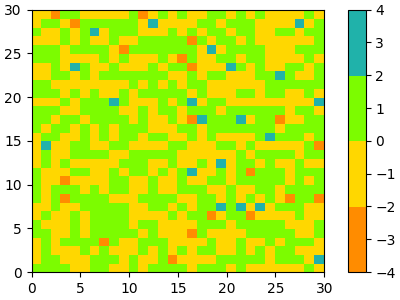In fact, that list may contain any valid matplotlib color specification. Particularly useful for creating custom colormaps are Nx4 numpy arrays. Because with the variety of numpy operations that we can do on a such an array, carpentry of new colormaps from existing colormaps become quite straight forward.

For example, suppose we want to make the first 25 entries of a 256-length "viridis" colormap pink for some reason:

viridis = cm.get_cmap('viridis', 256)
newcolors = viridis(np.linspace(0, 1, 256))
pink = np.array([248/256, 24/256, 148/256, 1])
newcolors[:25, :] = pink
newcmp = ListedColormap(newcolors)

plot_examples([viridis, newcmp])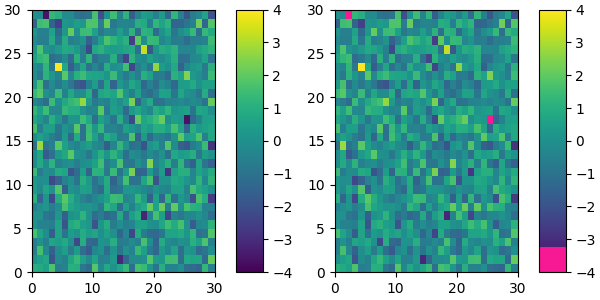We can easily reduce the dynamic range of a colormap; here we choose the middle 0.5 of the colormap. However, we need to interpolate from a larger colormap, otherwise the new colormap will have repeated values.

viridis_big = cm.get_cmap('viridis', 512)
newcmp = ListedColormap(viridis_big(np.linspace(0.25, 0.75, 256)))
plot_examples([viridis, newcmp])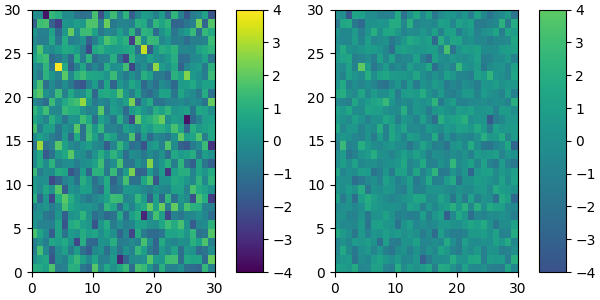and we can easily concatenate two colormaps:

top = cm.get_cmap('Oranges_r', 128)
bottom = cm.get_cmap('Blues', 128)

newcolors = np.vstack((top(np.linspace(0, 1, 128)),
bottom(np.linspace(0, 1, 128))))
newcmp = ListedColormap(newcolors, name='OrangeBlue')
plot_examples([viridis, newcmp])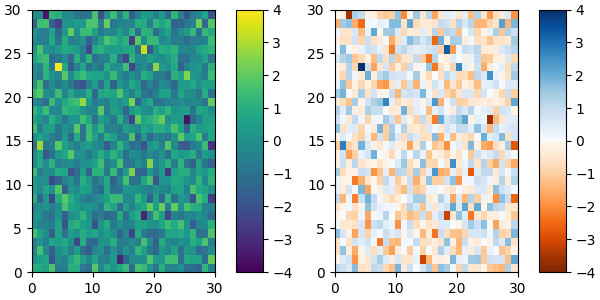Of course we need not start from a named colormap, we just need to create the Nx4 array to pass to ListedColormap. Here we create a colormap that goes from brown (RGB: 90, 40, 40) to white (RGB: 255, 255, 255).

N = 256
vals = np.ones((N, 4))
vals[:, 0] = np.linspace(90/256, 1, N)
vals[:, 1] = np.linspace(40/256, 1, N)
vals[:, 2] = np.linspace(40/256, 1, N)
newcmp = ListedColormap(vals)
plot_examples([viridis, newcmp])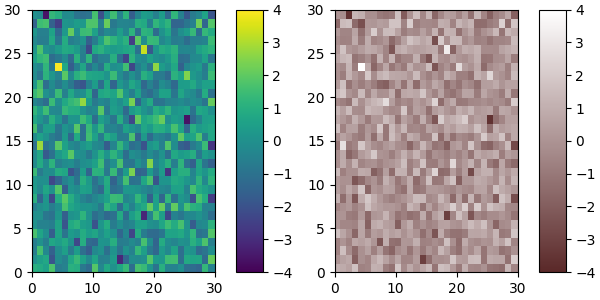## Creating linear segmented colormaps¶

LinearSegmentedColormap class specifies colormaps using anchor points between which RGB(A) values are interpolated.

The format to specify these colormaps allows discontinuities at the anchor points. Each anchor point is specified as a row in a matrix of the form [x[i] yleft[i] yright[i]], where x[i] is the anchor, and yleft[i] and yright[i] are the values of the color on either side of the anchor point.

If there are no discontinuities, then yleft[i]=yright[i]:

cdict = {'red':   [[0.0,  0.0, 0.0],
[0.5,  1.0, 1.0],
[1.0,  1.0, 1.0]],
'green': [[0.0,  0.0, 0.0],
[0.25, 0.0, 0.0],
[0.75, 1.0, 1.0],
[1.0,  1.0, 1.0]],
'blue':  [[0.0,  0.0, 0.0],
[0.5,  0.0, 0.0],
[1.0,  1.0, 1.0]]}

def plot_linearmap(cdict):
newcmp = LinearSegmentedColormap('testCmap', segmentdata=cdict, N=256)
rgba = newcmp(np.linspace(0, 1, 256))
fig, ax = plt.subplots(figsize=(4, 3), constrained_layout=True)
col = ['r', 'g', 'b']
for xx in [0.25, 0.5, 0.75]:
ax.axvline(xx, color='0.7', linestyle='--')
for i in range(3):
ax.plot(np.arange(256)/256, rgba[:, i], color=col[i])
ax.set_xlabel('index')
ax.set_ylabel('RGB')
plt.show()

plot_linearmap(cdict)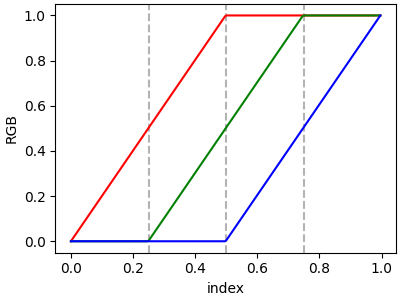In order to make a discontinuity at an anchor point, the third column is different than the second. The matrix for each of "red", "green", "blue", and optionally "alpha" is set up as:

cdict['red'] = [...
[x[i]      yleft[i]     yright[i]],
[x[i+1]    yleft[i+1]   yright[i+1]],
...]


and for values passed to the colormap between x[i] and x[i+1], the interpolation is between yright[i] and yleft[i+1].

In the example below there is a discontinuity in red at 0.5. The interpolation between 0 and 0.5 goes from 0.3 to 1, and between 0.5 and 1 it goes from 0.9 to 1. Note that red[0, 1], and red[2, 2] are both superfluous to the interpolation because red[0, 1] is the value to the left of 0, and red[2, 2] is the value to the right of 1.0.

cdict['red'] = [[0.0,  0.0, 0.3],
[0.5,  1.0, 0.9],
[1.0,  1.0, 1.0]]
plot_linearmap(cdict)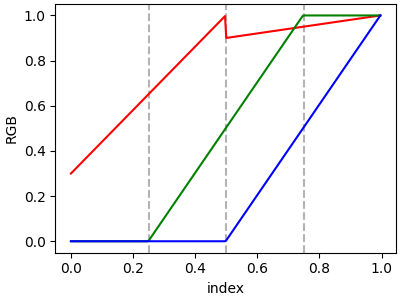### Directly creating a segmented colormap from a list¶

The above described is a very versatile approach, but admittedly a bit cumbersome to implement. For some basic cases, the use of LinearSegmentedColormap.from_list may be easier. This creates a segmented colormap with equal spacings from a supplied list of colors.

colors = ["darkorange", "gold", "lawngreen", "lightseagreen"]
cmap1 = LinearSegmentedColormap.from_list("mycmap", colors)


If desired, the nodes of the colormap can be given as numbers between 0 and 1. E.g. one could have the reddish part take more space in the colormap.

nodes = [0.0, 0.4, 0.8, 1.0]
cmap2 = LinearSegmentedColormap.from_list("mycmap", list(zip(nodes, colors)))

plot_examples([cmap1, cmap2])